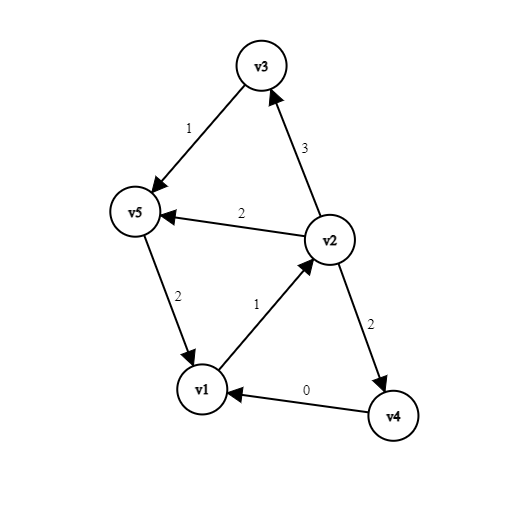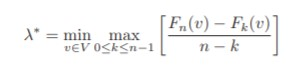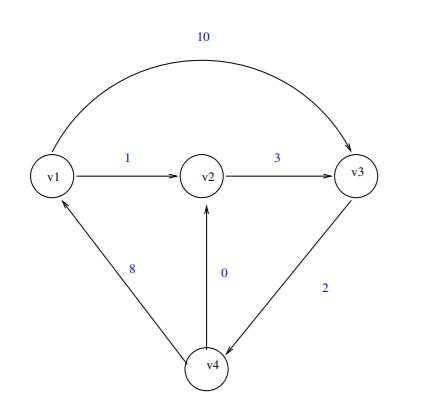×

Search anything:

# Karp's Minimum Mean Cycle Algorithm

#### Algorithms Graph Algorithms Dynamic Programming (DP)Get this book -> Problems on Array: For Interviews and Competitive Programming

In this article, we have presented Karp's Minimum Mean Cycle Algorithm along with C++ Implementation. Karp's theorem and Complexity Analysis.

1. Problem statement with example
2. Theorem given by Karp
3. Karp's Minimum Mean Cycle Algorithm
4. Implementation
5. Complexity Analysis
6. References

# Problem statement with example

In 1978 , Richard M. Karp gave a characterization of Minimum Mean Cycle. Given a strongly connected digraph, G(V,E) with n vertices consisting of non-negative weights. Mean Weight of a directed cycle defined as summation of edge weights of all edges present in a cycles over number of edges present in a cycle. Our task is to find Minimum Mean Cycle over all directed cycles present in a digraph.

### Example :In a given directed graph, 3 directed cycles are possible and their following mean weight are as shown :

Cycles Path Mean Weight
C1 v1->v2-v4->v1 (1+2+0)/3
C2 v1->v2->v5->v1 (1+2+2)/3
C3 v1->v2->v3->v5->v1 (1+3+1+2)/4
Our task is to find a directed cycle with minimum mean weight over all directed cycles. For a given graph,it comes out to be for Cycle C1 i.e 1.

# Theorem given by Karp

Let s be an arbitrary start vertex of G and λ* be Minimum Mean Weight over all all directed cycles.For each vertex v ∈ V and non-negative integer k , let Fk(v) be the minimum weight of any edge progression of length exactly k from s to v, of ∞ if no such edge progression exists. λ* can be defined asProof
It can be observed that if each edge weight is reduced by constant c then λ* is also reduced by c. RHS of above equation is also reduced by c as F(v) is reduced by c and Fk(v) reduced by k*c. Let translation constant be such that λ* becomes zero.

If λ*= 0 then (Fn(v) - Fk(v))/(n-k) = 0 for some value of v and k. It is known that graph doesn't consists of negative edges and there exists a cycle of weight zero . Hence , we can say that there is an edge progression of edge length k less than n ,from vertex s to v having minimum-mean weight,Fn(v) >= min0<=k<n Fk(v). It implies that max0<=k<n(Fn(v) - Fk(v)) >= 0 . On dividing n-k on both sides, it can be deduced to max0<=k<n(Fn(v) - Fk(v))/(n-k)>= 0.
Above condition λ*= 0 holds if and only if Fn(v) = min0<=k<n Fk(v) for some vertex v which can be proved simply by assuming cycle C and repeating over and over until its path length becomes n and k.

For more details, refer to .

# Karp's Minimum Mean Cycle Algorithm

### Terminology

|E| = Number of edges present in a graph
|V| = n, Number of Vertices in a graph

### Steps:

1. Choose an arbitrary starting vertex s.
2. Compute the shortest path from vertex s to v having edge length k, where 0 < k <= |V|.
3. Store the computed value in step-2 in an 2-D array say 'dp' ,where dp[k][v] tells shortest length from vertex s to v having edge length k.
4. For every vertex v , calculate the Mean Weight using Karp's theorem, max((dp[n][v] - dp[k][v])/(n-k)) where 0<= k <= n-1
5. Store the results computed in step-4 in an 1D-array say 'mean'.
6. Minimum Mean is the minimum value in the array 'mean'.

#### How to compute the shortest path?

Dynamic programming can be implemented to find shortest path from vertex s to vertex v containing k edges contains optimal sub-structure and overlapping sub-problems.
`````` Recursive Solution
dp[k][v] = min(dp[k][v], dp[k-1][u] + weight(u,v))

For each k in range(0,n), we compute above relation for every vertex v in range(0,n) and store it in dp[k][v]. Here, W(u,v) is a weight of direct edge between vertex u and v.
``````

# Implementation

Implementation in C++:

``````#include<bits/stdc++.h>
using namespace std;

//represents number of edges
int N;

//represents an edge
typedef struct{
int from,weight;
}edge;

vector<edge> edges[N];

edges[to].push_back({from,weight});
}

//computes the shortest Path
void shortestpath(int dp[][N]){

//intialize all distances to -1
for(int i=0; i<=N; i++){
for(int j=0; j<N; j++)
dp[i][j]= -1;
}

//starting vertex to be first vertex 0
dp= 0;

//for all path length
for(int k=1; k<=N; k++){

//for every vertex
for(int v=0; v<N; v++){
//direct edges (u,v)
for(int u=0; u<edges[v].size(); u++){

if(dp[k-1][edges[u].from] == -1)
continue;

int weight_u_to_v=edges[v][u].weight;

if(dp[k][v]==-1)
dp[k][v]= dp[k-1][edges[u].from] + weight_u_to_v;
else
dp[k][v] = min(dp[k][v], dp[k-1][edges[u].from] + weight_u_to_v);
}
}
}

}

//computes minimum mean weight of cycle in graph
double minMeanWeight(){

int dp[N+1][N];

shortestpath(dp);

//stores mean values
double mean[N];
for(int i=0;i<N;i++)
avg[i]=-1;

//Applying Karp's Theorem
for(int i=0;i<N;i++){

if(dp[N][i]==-1)
continue;

for(int k=0;k<N;k++){

if(dp[k][i] != -1)
mean[i] = max(mean[i],((double)dp[N][i]-dp[k][i])/(N-k));

}
}

//computes minimum value from mean[]
double minWeight = mean;
for(int i=0;i<N;i++){
if(mean[i] != -1)
minWeight = min(minWeight,mean[i]);
}
return minWeight;
}
int main(){
N=4;

cout<<minMeanWeight()<<endl;

return 0;
}
``````

Input:Table:
A Table could be generated for following approach, where each entry (i,j) represents a value stored in dp[i][j], 0<=i, j<|V|.
(k,v) 0 1 2 3 4 max((dp[n][v] - dp[k][v])/(n-k))
v1 0 -1 -1 20 14 7/2
v2 -1 1 -1 12 6 5/3
v3 -1 10 4 -1 15 11/2
v4 -1 -1 12 6 -1 -1

Output:

``````1.66667
``````

# Complexity Analysis

• Time Complexity : O(|V| * |E|), for computation of shortest path using dynamic programming.
• Auxiliary Space Complexity: O(|V| * |E| + |V|), for DP matrix and storing mean weights of every path length

With this article at OpenGenus, you must have a strong idea of Karp's Minimum Mean Cycle Algorithm.

1. R. M. Karp, A characterization of the minimum cycle mean in a digraph,Discrete mathematics 23 (3) (1978) 309–311
2. Introduction to Algorithms - Problem Set 9 Solutions by Massachusetts Institute of Technology courses.csail.mit.edu/6.046/fall01/handouts/ps9sol.pdf